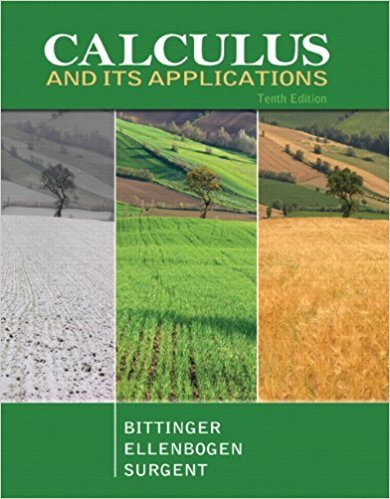×
×

# Solutions for Chapter 1.3: Average Rates of Change## Full solutions for Calculus and Its Applications | 10th Edition

ISBN: 9780321694331Solutions for Chapter 1.3: Average Rates of Change

Solutions for Chapter 1.3
4 5 0 271 Reviews
28
4
##### ISBN: 9780321694331

Since 56 problems in chapter 1.3: Average Rates of Change have been answered, more than 23943 students have viewed full step-by-step solutions from this chapter. This expansive textbook survival guide covers the following chapters and their solutions. Calculus and Its Applications was written by and is associated to the ISBN: 9780321694331. This textbook survival guide was created for the textbook: Calculus and Its Applications, edition: 10. Chapter 1.3: Average Rates of Change includes 56 full step-by-step solutions.

Key Calculus Terms and definitions covered in this textbook
• Binomial theorem

A theorem that gives an expansion formula for (a + b)n

• Conversion factor

A ratio equal to 1, used for unit conversion

• Coordinate plane

See Cartesian coordinate system.

• equation of a quadratic function

ƒ(x) = ax 2 + bx + c(a ? 0)

• Explanatory variable

A variable that affects a response variable.

• Frequency (in statistics)

The number of individuals or observations with a certain characteristic.

• Grapher or graphing utility

Graphing calculator or a computer with graphing software.

• Linear regression

A procedure for finding the straight line that is the best fit for the data

• Linear system

A system of linear equations

• Logarithmic regression

See Natural logarithmic regression

• Multiplicative inverse of a matrix

See Inverse of a matrix

• nth root of a complex number z

A complex number v such that vn = z

• Parametrization

A set of parametric equations for a curve.

• Positive numbers

Real numbers shown to the right of the origin on a number line.

A function that can be written in the form ƒ(x) = ax 2 + bx + c, where a, b, and c are real numbers, and a ? 0.

• Quartic regression

A procedure for fitting a quartic function to a set of data.

• Quartile

The first quartile is the median of the lower half of a set of data, the second quartile is the median, and the third quartile is the median of the upper half of the data.

• Vector equation for a line in space

The line through P0(x 0, y0, z0) in the direction of the nonzero vector V = <a, b, c> has vector equation r = r0 + tv , where r = <x,y,z>.

• Velocity

A vector that specifies the motion of an object in terms of its speed and direction.

• Vertical component

See Component form of a vector.

×